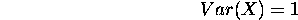Next: Initialization Up: Data preprocessing Previous: Data encoding

### Scaling

It is common that the components of the input data are scaled to have unit variance.This can be achieved by dividing the components by the square roots of their corresponding variances. This assures that for each component, the difference between two samples contribute approximately an equal amount to the summed distance measure between an input sample and codebook vector.

Because the similarity measure usually loses identity of component differences via a summation, or treats all components equally, the components must contribute approximately as much to the similarity measure. Otherwise, a component with large variance would shadow components with small variance and thus only the components with large variance would contribute to the distance measure used as a similarity measure.

Jaakko Hollmen
Fri Mar 8 13:44:32 EET 1996